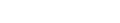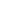xToughnessEncyclopedia
In materials science
Materials science
Materials science is an interdisciplinary field applying the properties of matter to various areas of science and engineering. This scientific field investigates the relationship between the structure of materials at atomic or molecular scales and their macroscopic properties. It incorporates...

and metallurgy
Metallurgy
Metallurgy is a domain of materials science that studies the physical and chemical behavior of metallic elements, their intermetallic compounds, and their mixtures, which are called alloys. It is also the technology of metals: the way in which science is applied to their practical use...

, toughness is the ability of a material to absorb energy and plastically deform without fracturing; Material toughness is defined as the amount of energy
Energy
In physics, energy is an indirectly observed quantity. It is often understood as the ability a physical system has to do work on other physical systems...

per volume that a material can absorb before rupturing. It is also defined as the resistance to fracture
Fracture
A fracture is the separation of an object or material into two, or more, pieces under the action of stress.The word fracture is often applied to bones of living creatures , or to crystals or crystalline materials, such as gemstones or metal...

of a material when stressed
Stress (physics)
In continuum mechanics, stress is a measure of the internal forces acting within a deformable body. Quantitatively, it is a measure of the average force per unit area of a surface within the body on which internal forces act. These internal forces are a reaction to external forces applied on the body...

.

Toughness requires a balance of strength
Strength of materials
In materials science, the strength of a material is its ability to withstand an applied stress without failure. The applied stress may be tensile, compressive, or shear. Strength of materials is a subject which deals with loads, deformations and the forces acting on a material. A load applied to a...

and ductility
Ductility
In materials science, ductility is a solid material's ability to deform under tensile stress; this is often characterized by the material's ability to be stretched into a wire. Malleability, a similar property, is a material's ability to deform under compressive stress; this is often characterized...

.

## Mathematical definition

Toughness can be determined by measuring the area (i.e., by taking the integral) underneath the stress-strain curve
Stress-strain curve
During tensile testing of a material sample, the stress–strain curve is a graphical representation of the relationship between stress, derived from measuring the load applied on the sample, and strain, derived from measuring the deformation of the sample, i.e. elongation, compression, or distortion...

and its energy of mechanical deformation per unit volume prior to fracture. The explicit mathematical description is:Where
•is strain
•is the strain upon failure
•is stress

Another definition is the ability to absorb mechanical (or kinetic) energy up to failure.
The area covered under stress strain curve is called toughness.

If the upper limit of integration up to the yield point is restricted, then the energy absorbed per unit volume is known as the modulus of resilience
Resilience
Resilience is the property of a material to absorb energy when it is deformed elastically and then, upon unloading to have this energy recovered. In other words, it is the maximum energy per unit volume that can be elastically stored...

.
Mathematically, the modulus of resilience can be expressed by the product of the square of the yield strain divided by two times the Young's modulus.

## Toughness tests

Tests can be done by using a pendulum and basic physics to measure how much energy it will hold when released from a particular height. By having a sample at the bottom of its swing, a measure of toughness can be found, as in the Charpy
Charpy impact test
The Charpy impact test, also known as the Charpy v-notch test, is a standardized high strain-rate test which determines the amount of energy absorbed by a material during fracture. This absorbed energy is a measure of a given material's toughness and acts as a tool to study temperature-dependent...

and Izod impact tests
Izod impact strength test
Izod impact strength testing is an ASTM standard method of determining impact strength. A notched sample is generally used to determine impact strength....

.

## Unit of toughness

Toughness is measured in units of joule
Joule
The joule ; symbol J) is a derived unit of energy or work in the International System of Units. It is equal to the energy expended in applying a force of one newton through a distance of one metre , or in passing an electric current of one ampere through a resistance of one ohm for one second...

s per square metre
Square metre
The square metre or square meter is the SI derived unit of area, with symbol m2 . It is defined as the area of a square whose sides measure exactly one metre...

(J/m2) in the SI
Si
Si, si, or SI may refer to :- Measurement, mathematics and science :* International System of Units , the modern international standard version of the metric system...

system and inch-pound-force
Pound-force
The pound force is a unit of force in some systems of measurement including English engineering units and British gravitational units.- Definitions :...

per cubic inch
Cubic inch
The cubic inch is a unit of measurement for volume in the Imperial units and United States customary units systems. It is the volume of a cube with each of its 3 sides being one inch long....

(in·lbf/in3) in US customary units.

## Toughness and strength

Strength
Strength of materials
In materials science, the strength of a material is its ability to withstand an applied stress without failure. The applied stress may be tensile, compressive, or shear. Strength of materials is a subject which deals with loads, deformations and the forces acting on a material. A load applied to a...

and toughness are related. A material may be strong and tough if it ruptures under high forces, exhibiting high strains, while brittle materials may be strong but with limited strain values so that they are not tough. Generally speaking, strength indicates how much force the material can support, while toughness indicates how much energy a material can absorb before rupturing.

• Fracture toughness
Fracture toughness
In materials science, fracture toughness is a property which describes the ability of a material containing a crack to resist fracture, and is one of the most important properties of any material for virtually all design applications. The fracture toughness of a material is determined from the...

• Graph toughness
Graph toughness
In graph theory, toughness is a measure of the connectivity of a graph. A graph is said to be -tough for a given real number if, for every integer , cannot be split into different connected components by the removal of fewer than vertices...

• Hardness
• Impact (mechanics)
• Resilience
Resilience
Resilience is the property of a material to absorb energy when it is deformed elastically and then, upon unloading to have this energy recovered. In other words, it is the maximum energy per unit volume that can be elastically stored...

• Shock (mechanics)
Shock (mechanics)
A mechanical or physical shock is a sudden acceleration or deceleration caused, for example, by impact, drop, kick, earthquake, or explosion. Shock is a transient physical excitation....